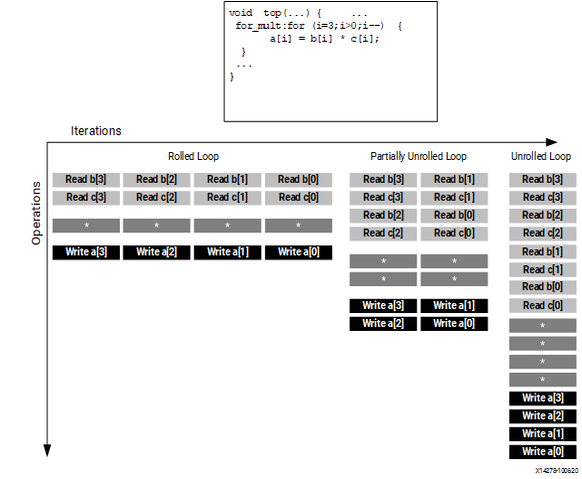Unrolling Loops to Improve Pipelining - 2021.2 English

Vitis High-Level Synthesis User Guide (UG1399)

Document ID
UG1399
ft:locale
English (United States)
Release Date
2021-12-15
Version
2021.2 English

By default, loops are kept rolled in Vitis HLS. These rolled loops generate a hardware resource which is used by each iteration of the loop. While this creates a resource efficient block, it can sometimes be a performance bottleneck.

Vitis HLS provides the ability to unroll or partially unroll FOR loops using the UNROLL pragma or directive.

The following figure shows both the advantages of loop unrolling and the implications that must be considered when unrolling loops. This example assumes the arrays a[i], b[i], and c[i] are mapped to block RAMs. This example shows how easy it is to create many different implementations by the simple application of loop unrolling.

Figure 1. Loop Unrolling DetailsRolled Loop
When the loop is rolled, each iteration is performed in separate clock cycles. This implementation takes four clock cycles, only requires one multiplier and each block RAM can be a single-port block RAM.
Partially Unrolled Loop
In this example, the loop is partially unrolled by a factor of 2. This implementation required two multipliers and dual-port RAMs to support two reads or writes to each RAM in the same clock cycle. This implementation does however only take 2 clock cycles to complete: half the initiation interval and half the latency of the rolled loop version.
Unrolled loop
In the fully unrolled version all loop operation can be performed in a single clock cycle. This implementation however requires four multipliers. More importantly, this implementation requires the ability to perform 4 reads and 4 write operations in the same clock cycle. Because a block RAM only has a maximum of two ports, this implementation requires the arrays be partitioned.

To perform loop unrolling, you can apply the UNROLL directives to individual loops in the design. Alternatively, you can apply the UNROLL directive to a function, which unrolls all loops within the scope of the function.

If a loop is completely unrolled, all operations will be performed in parallel if data dependencies and resources allow. If operations in one iteration of the loop require the result from a previous iteration, they cannot execute in parallel but will execute as soon as the data is available. A completely unrolled and fully optimized loop will generally involve multiple copies of the logic in the loop body.

The following example code demonstrates how loop unrolling can be used to create an optimized design. In this example, the data is stored in the arrays as interleaved channels. If the loop is pipelined with II=1, each channel is only read and written every eighth block cycle.

// Array Order :  0  1  2  3  4  5  6  7  8     9     10    etc. 16       etc...
// Sample Order:  A0 B0 C0 D0 E0 F0 G0 H0 A1    B1    C2    etc. A2       etc...
// Output Order:  A0 B0 C0 D0 E0 F0 G0 H0 A0+A1 B0+B1 C0+C2 etc. A0+A1+A2 etc...

#define CHANNELS 8
#define SAMPLES  400
#define N CHANNELS * SAMPLES

void foo (dout_t d_out[N], din_t d_in[N]) {
int i, rem;

// Store accumulated data
static dacc_t acc[CHANNELS];

// Accumulate each channel
For_Loop: for (i=0;i<N;i++) {
rem=i%CHANNELS;
acc[rem] = acc[rem] + d_in[i];
d_out[i] = acc[rem];
}
}

Partially unrolling the loop by a factor of 8 will allow each of the channels (every eighth sample) to be processed in parallel (if the input and output arrays are also partitioned in a cyclic manner to allow multiple accesses per clock cycle). If the loop is also pipelined with the rewind option, this design will continuously process all 8 channels in parallel if called in a pipelined fashion (that is, either at the top, or within a dataflow region).

void foo (dout_t d_out[N], din_t d_in[N]) {
#pragma HLS ARRAY_PARTITION variable=d_i type=cyclic factor=8 dim=1
#pragma HLS ARRAY_PARTITION variable=d_o type=cyclic factor=8 dim=1

int i, rem;

// Store accumulated data
static dacc_t acc[CHANNELS];

// Accumulate each channel
For_Loop: for (i=0;i<N;i++) {
#pragma HLS PIPELINE rewind
#pragma HLS UNROLL factor=8

rem=i%CHANNELS;
acc[rem] = acc[rem] + d_in[i];
d_out[i] = acc[rem];
}
}

Partial loop unrolling does not require the unroll factor to be an integer multiple of the maximum iteration count. Vitis HLS adds an exit check to ensure partially unrolled loops are functionally identical to the original loop. For example, given the following code:

for(int i = 0; i < N; i++) {
a[i] = b[i] + c[i];
}

Loop unrolling by a factor of 2 effectively transforms the code to look like the following example where the break construct is used to ensure the functionality remains the same:

for(int i = 0; i < N; i += 2) {
a[i] = b[i] + c[i];
if (i+1 >= N) break;
a[i+1] = b[i+1] + c[i+1];
}

Because N is a variable, Vitis HLS might not be able to determine its maximum value (it could be driven from an input port). If the unrolling factor, which is 2 in this case, is an integer factor of the maximum iteration count N, the skip_exit_check option removes the exit check and associated logic. The effect of unrolling can now be represented as:

for(int i = 0; i < N; i += 2) {
a[i] = b[i] + c[i];
a[i+1] = b[i+1] + c[i+1];
}

This helps minimize the area and simplify the control logic.